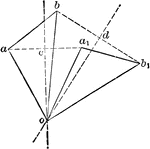### Periodic Center of Motion

Illustration showing the periodic center of motion as it often happens when two positions of a line…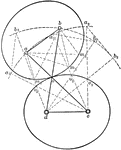### Centroid

Illustration showing a centroid, "the curve passing through the successive positions of the instantaneous…### Motion of Open Curves

"Since these curves are not closed, one pair cannot be used for continuous motion; but a pair of such…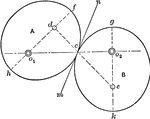### Rolling of Equal Ellipses

"If two equal ellipses, each turning about one of its foci, are placed in contact in such a way that…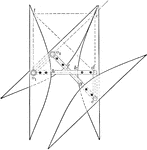### Rolling of Equal Hyperbolas

Illustration of the rolling of equal hyperbolas. If two equal hyperbolas are placed so that the distances…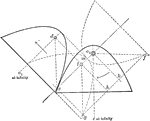### Rolling of Equal Parabolas

Illustration of the rolling of equal parabolas. "The two parabolas may be considered as two ellipses…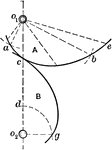### Construction of Logarithmic Spiral

Illustration showing how a rolling logarithmic spiral can be constructed by taking successive values…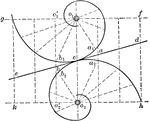### Rolling of Logarithmic Spirals

Illustration showing the rolling of two logarithmic spirals of equal obliquity.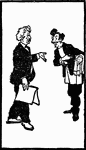### Mark Twain Speaking Italian

Mark Twain saying "Sono Dispiacentissimo" to an Italian servant.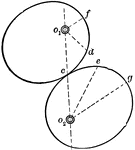### Rolling of Non-cylindrical Surfaces

Illustration showing that the rolling of non-cylindrical surfaces. "If the angular velocity ratio of…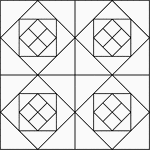### Geometric Block Pattern 1

Geometric pattern for translation and rotation exercises.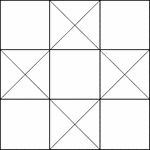### Geometric Block Pattern 10

Geometric pattern for translation and rotation exercises.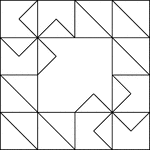### Geometric Block Pattern 100

Geometric pattern for translation and rotation exercises.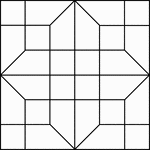### Geometric Block Pattern 101

Geometric pattern for translation and rotation exercises.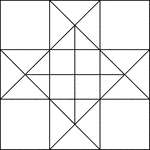### Geometric Block Pattern 11

Geometric pattern for translation and rotation exercises.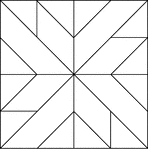### Geometric Block Pattern 12

Geometric pattern for translation and rotation exercises.### Geometric Block Pattern 13

Geometric pattern for translation and rotation exercises.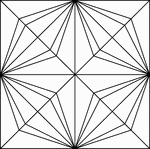### Geometric Block Pattern 14

Geometric pattern for translation and rotation exercises.### Geometric Block Pattern 15

Geometric pattern for translation and rotation exercises.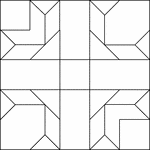### Geometric Block Pattern 16

Geometric pattern for translation and rotation exercises.### Geometric Block Pattern 17

Geometric pattern for translation and rotation exercises.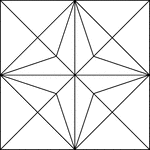### Geometric Block Pattern 18

Geometric pattern for translation and rotation exercises.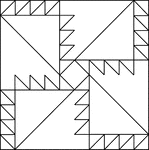### Geometric Block Pattern 19

Geometric pattern for translation and rotation exercises.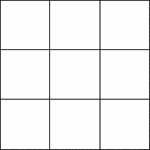### Geometric Block Pattern 2

Geometric pattern for translation and rotation exercises.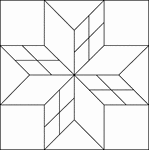### Geometric Block Pattern 20

Geometric pattern for translation and rotation exercises.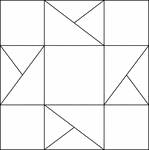### Geometric Block Pattern 21

Geometric pattern for translation and rotation exercises.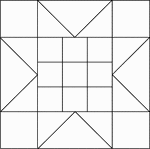### Geometric Block Pattern 22

Geometric pattern for translation and rotation exercises.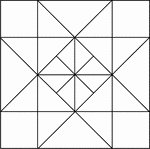### Geometric Block Pattern 23

Geometric pattern for translation and rotation exercises.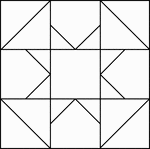### Geometric Block Pattern 24

Geometric pattern for translation and rotation exercises.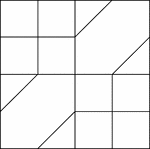### Geometric Block Pattern 25

Geometric pattern for translation and rotation exercises.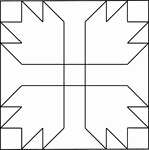### Geometric Block Pattern 26

Geometric pattern for translation and rotation exercises.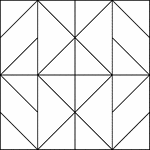### Geometric Block Pattern 27

Geometric pattern for translation and rotation exercises.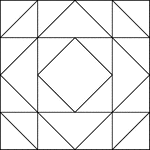### Geometric Block Pattern 28

Geometric pattern for translation and rotation exercises.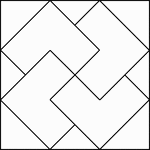### Geometric Block Pattern 29

Geometric pattern for translation and rotation exercises.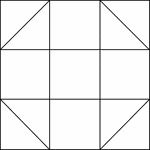### Geometric Block Pattern 3

Geometric pattern for translation and rotation exercises.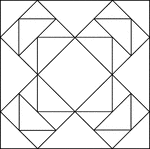### Geometric Block Pattern 30

Geometric pattern for translation and rotation exercises.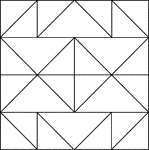### Geometric Block Pattern 31

Geometric pattern for translation and rotation exercises.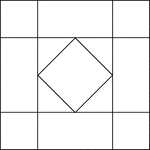### Geometric Block Pattern 32

Geometric pattern for translation and rotation exercises.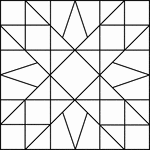### Geometric Block Pattern 33

Geometric pattern for translation and rotation exercises.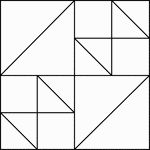### Geometric Block Pattern 34

Geometric pattern for translation and rotation exercises.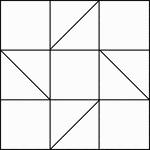### Geometric Block Pattern 35

Geometric pattern for translation and rotation exercises.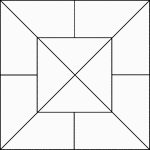### Geometric Block Pattern 36

Geometric pattern for translation and rotation exercises.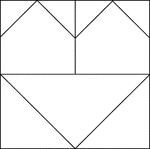### Geometric Block Pattern 37

Geometric pattern for translation and rotation exercises.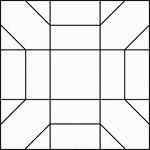### Geometric Block Pattern 38

Geometric pattern for translation and rotation exercises.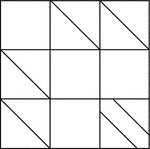### Geometric Block Pattern 39

Geometric pattern for translation and rotation exercises.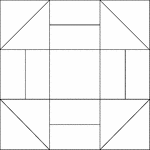### Geometric Block Pattern 4

Geometric pattern for translation and rotation exercises.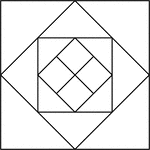### Geometric Block Pattern 40

Geometric pattern for translation and rotation exercises.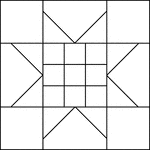### Geometric Block Pattern 41

Geometric pattern for translation and rotation exercises.### Geometric Block Pattern 42

Geometric pattern for translation and rotation exercises.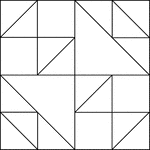### Geometric Block Pattern 43

Geometric pattern for translation and rotation exercises.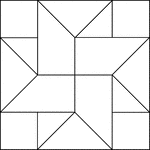### Geometric Block Pattern 44

Geometric pattern for translation and rotation exercises.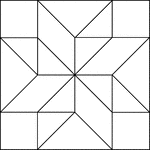### Geometric Block Pattern 45

Geometric pattern for translation and rotation exercises.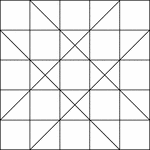### Geometric Block Pattern 46

Geometric pattern for translation and rotation exercises.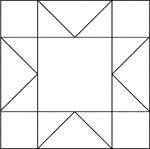### Geometric Block Pattern 47

Geometric pattern for translation and rotation exercises.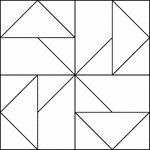### Geometric Block Pattern 48

Geometric pattern for translation and rotation exercises.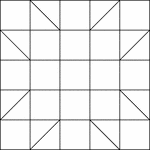### Geometric Block Pattern 49

Geometric pattern for translation and rotation exercises.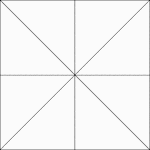### Geometric Block Pattern 5

Geometric pattern for translation and rotation exercises.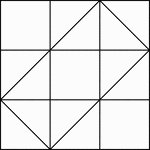### Geometric Block Pattern 50

Geometric pattern for translation and rotation exercises.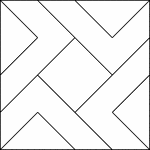### Geometric Block Pattern 51

Geometric pattern for translation and rotation exercises.